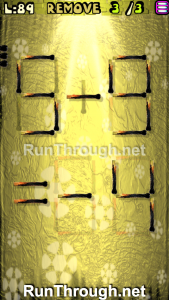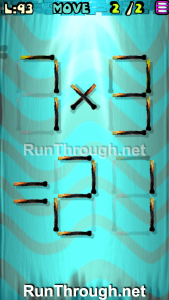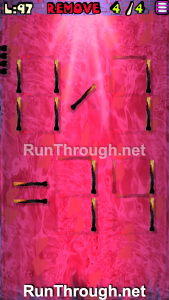# Move the Matches Puzzles Episode 12 Levels 81-100 Walkthrough

Walkthrough for all the solutions and answers to the game Move the Matches Puzzles by K-Lab. Get the app on the Google Play Store to play along if you do not already have it. This is for episode 12, levels 81 through 100.

For the walkthrough (or answer) images, we will be showing the final image and answer of the Matches Puzzle. This will allow you to see where everything goes, and to know for sure that the puzzle works.  These puzzles have been recently updated, so other walkthroughs do not have the updated puzzles, but we try to always stay up to date with every walkthrough and answer!

### Level 81Instructions: Move 2 matches to correct the equation

Solution: 7-5=2

### Level 82Instructions: Move 1 match to correct the equation

Solution: 1-9=-8

### Level 83Instructions: Remove 4 matches to correct the equation

Solution: 2+5=7

### Level 84Instructions: Add 4 matches to correct the equation

Solution: 1×6=06

### Level 85Instructions: Move 3 matches to correct the equation

Solution: 9/3=3

### Level 86Instructions: Remove 4 matches to correct the equation

Solution: 4+7=11

### Level 87Instructions: Move 1 match to correct the equation

Solution: 6+9=15

### Level 88Instructions: Add 4 matches to correct the equation

Solution: 3-8=-5

### Level 89Instructions: Remove 3 matches to correct the equation

Solution: 5-9=-4

### Level 90Instructions: Move 2 matches to get 4 triangles

### Level 91Instructions: Move 1 match to correct the equation

Solution: 11×4=44

### Level 92Instructions: Move 1 match to correct the equation

Solution: 9/9=1

### Level 93Instructions: Move 2 matches to correct the equation

Solution: 7×3=21

### Level 94Instructions: Add 4 matches to correct the equation

Solution: 5×9=45

### Level 95Instructions: Move 1 match to correct the equation

Solution: 5-9=-4

### Level 96Instructions: Move 3 matches to correct the equation

Solution: 9+5=14

### Level 97Instructions: Remove 4 matches to correct the equation

Solution: 11/1=11

### Level 98Instructions: Move 4 matches to get 5 squares

### Level 99Instructions: Move 3 matches to correct the equation

Solution: 5×9=45

### Level 100Instructions: Move 3 matches to get 4 squares